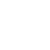# Interfacing PIC microcontroller with LM335 sensor | MPLAB Projects

LM335 Sensor is a 3-pin analog device which can measure temperature (converts temperature to analog voltage). This sensor requires an ADC to convert the analog data into digital data.
This post shows how to build a simple thermometer using PIC12F1822 microcontroller and LM335 analog temperature sensor. Temperature data is displayed on 16×2 LCD screen (I2C LCD). This project works also with DFRobot I2C LCD displays.

The compiler used in this project is Microchip MPLAB XC8 (MPLAB X IDE with MPLAB XC8 compiler).

The LM335 sensor has the following features (from LM335 datasheet):

• Directly Calibrated to the Kelvin Temperature Scale
• 1°C Initial Accuracy Available
• Operates from 400 μA to 5 mA
• Less than 1-Ω Dynamic Impedance
• Easily Calibrated
• Wide Operating Temperature Range
• 200°C Overrange
• Low Cost

The LM335 has a breakdown voltage directly proportional to absolute temperature at 10 mV/°K. For example if the LM335 output voltage is equal to 3.03 (3030 mV) that means the temperature is: 303 Kelvin = 30 °Celsius.

The PIC12F1822 is an 8-bit microcontroller which has 4 analog channels with 10-bit resolution. The good thing with this microcontroller is the fixed voltage reference. With the fixed voltage reference we get approximately an exact result. Normally negative and positive references of the ADC module are VSS and VDD, but VDD is not exactly equal to 5.00V and here we should use the fixed voltage reference as a positive reference of the ADC module.

The PIC12F1822 has 3 fixed voltage references: 1.024V, 2.048V and 4.096V. For example if we set the fixed voltage reference to 4.096V and the ADC module is configured so that the negative and the positive references are VSS and FVR (Fixed Voltage Reference) respectively, in this case the equivalent 10-bit digital value of 4.096V is 1023 and 3.00V is 3.00 * 1023/4.096 = 749 , and so on.
In this project I used 4.096 because the LM335 output is between 2.23V (temperature = -50°C) and 3.98V (temperature = +125°C).

Hardware Required:

• PIC12F1822 microcontroller  —  datasheet
• LM335 temperature sensor   —  datasheet
• 16×2 LCD screen
• PCF8574 I/O expander (or PCF8574A)   —   PCF8574 datasheet
• 5 x 10k ohm resistor
• 2.2k ohm resistor
• 330 ohm resistor
• 10k ohm variable resistor or potentiometer
• 5V source
• Jumper wires

Interfacing PIC microcontroller with LM335 sensor circuit:
Project circuit schematic diagram is shown below.(All grounded terminals are connected together)

The LM335 sensor has 3 pins (from left to right):
Pin 1 for calibration, not used in this example
Pin 2: output
Pin 3: GND (ground).

The output pin of the LM335 sensor is connected to analog channel 0 (RA0). I chose the 2.2K ohm because as written in the datasheet for optimum accuracy the current flows through the LM335 should be 1mA. For example if the temperature = 27°C, the output will be 3.00V and assume the supply voltage is exactly 5.00V that means the current flows through the sensor is ( 5 – 3)/2.2 = 0.90mA which is good enough. Also the value 2.2K is a standard value and well used.

In this project the PIC12F1822 microcontroller runs with its internal oscillator @ 8 MHz, MCLR pin is configured as an input pin.

Interfacing PIC microcontroller with LM335 sensor C code:
The C code below is for MPLAB XC8 compiler, it was tested with version 2.00 installed on MPLAB X IDE version 5.05 with an optimization level = 1 (Project Properties —> XC8 Compiler —> Optimization —> Optimization level).

RAM used: 95 bytes (74%)
ROM used: 1725 words (84%)

To be able to compile the C code, a small I2C LCD library for MPLAB XC8 compiler is required which can be downloaded from the following link:
I2C LCD driver for MPLAB XC8 compiler

The I2C LCD driver file is included with the line:
#include “I2C_LCD.c”

The hardware I2C module of the PIC12F1822 is initialized with a clock frequency of 100KHz (100000Hz):
I2C_Init(100000);

The I2C LCD is initialized with an I2C address of 0x4E:
LCD_Begin(0x4E);

The microcontroller used in this example is PIC12F1822, configuration words are:

Where:

• Fail-Safe Clock Monitor enabled
• Internal/External Switchover mode enabled
• CLKOUT function is disabled, I/O function on the CLKOUT pin
• Brown-out Reset (BOR) enabled
• Data memory code protection disabled
• Program memory code protection disabled
• RE3/MCLR pin function is digital input, MCLR internally tied to VDD
• Power-up Timer (PWRT) disabled
• Watchdog Timer (WDT) disabled
• INTOSCIO oscillator: I/O function on CLKIN pin
• Low voltage programming disabled
• In-Circuit Debugger disabled
• Brown-out Reset voltage (Vbor), low trip point selected
• Stack Overflow or Underflow will cause a Reset
• 4xPLL disabled
• Flash Program Memory Self Write disabled

MPLAB XC8 code:

### 3 thoughts on “Interfacing PIC microcontroller with LM335 sensor | MPLAB Projects”

1.Ömer Faruk Özcan

Thank you very much 🙂

2.Ömer Faruk Özcan

1.